# Yarn Count System and Determination by Different Methods

### Yarn Count System and Determination by Different Methods

Md. Didarul Islam
Dept. of Textile Engineering
BGMEA University of Fashion & Technology (BUFT), Dhaka
Email: 181-175-801@buft.edu.bd

Definition of Yarn Count:

Yarn count is a numerical expression which defines its fineness or coarseness. It also expresses weather the yarn is thick or thin. A definition is given by the textile institute – “Count is a number which indicates the mass per unit length or the length per unit mass of yarn.”

Types of Yarn Count:

Basically, there are mainly two ways of yarn count;

1. Direct count system
2. Indirect count System

Direct count system:

In a direct yarn counting system, the yarn number or count is the weight of a unit length of yarn. This means the higher the yarn count number, the heavier or thicker the yarn. It is fixed length system. This system is used for express the count of man-made fiber, jute, silk etc.

……………………………. W x l
Direct Count, N = —————
……………………………. w x L

Where,

N = The yarn number or, count
W = The weight of the sample
w = The unit weight of the sample
L = The length of the sample
l = The unit length of the sample

Tex, Denier, Pounds per spindle is the unit which is expressed in direct system.

Tex: The yarn number or count in the Tex system is the weight in grams of 1000m or 1 km of yarn.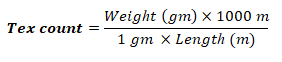Denier: The count of yarn in the denier system is the weight in grams of 9000m or 9 km of yarn.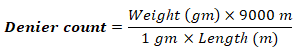Pound per Spindle: The yarn count in pounds per spindle is the weight in pounds of 14,400 yards of yarn. Jute count is expressed by this system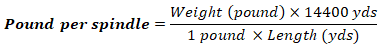Indirect count system:

In an indirect yarn counting system, the yarn number or count is the number of “units of length” per “unit of weight” of yarn. This means the higher the yarn number, the finer or thinner the yarn. It is based on the fixed weight system. This system is used for express the count of natural fiber.

………………………………. L x w
Indirect Count, N = ———————
………………………………. l x W

English count, Metric count, Worsted are expressed in indirect system.

English Count: It is defined as the number of hanks (840 yards) per pounds. It is denoted by Ne.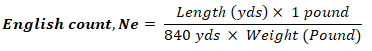Metric Count: It is defined as the number of hanks (1000 m) per kg. It is defined by Nm.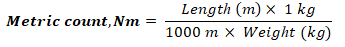Worsted Count: It is defined as the number of hanks (560 yards) per pounds.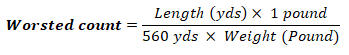Different Methods of Yarn Count Determination:

• Beesley Balance
• Wrap Reel Balance
• Stubb Yarn Balance

Determination of yarn count by Beesley Balance:

Beesley Balance: When a cotton yarn is supplied in short length or removed from fabric, the count of yarn can be measured by a special type of balance is called Beesley’s balance.

Procedure:

• At first collect denim sample.
• In this sample, the warp direction yarn color is blue and weft direction yarn color is white. First of all, 12 yarn withdrawn in warp direction from that fabric. Again 12 yarn withdrawn in weft direction.
• Marking all withdrawn yarn by template and cut them.
• Now placed that yarn in hook until the pointer comes in level with the datum line.
• At that stage the threads are taken out and counted which givers directly the count of yarn taken for testing.

Sample Attachment: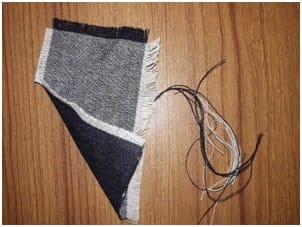Experimental data:

For warp: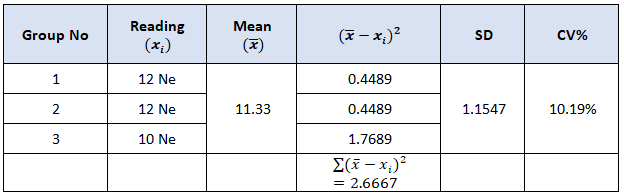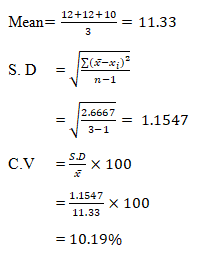For Weft: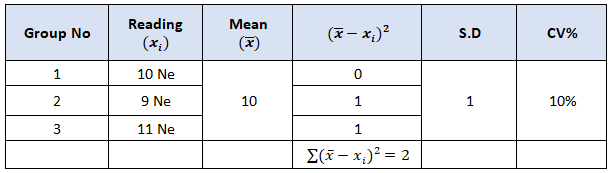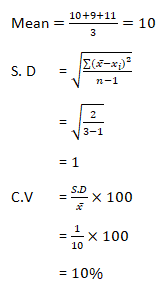Result Representation: 3 results were taken in the beesley balance m/c where the warp and weft yarn of the denim fabric was taken. We got 3 different result. After calculating the CV % (coefficient of variation ) we can say that the warp and weft yarns in our denim sample are quite different from each other.

Determination of yarn count by Wrap Reel Balance:

Wrap Reel Balance: A wrap reel is a device for measuring yarn and making it into hanks of a standard size. The reel is of a standard size and its revolutions are counted as the yarn is wrapped around it.

Procedure:

1. Make lea by Wrap reel.
2. Weight the lee by analytic balance.
3. Convert the weight gram to pound.
4. Now the data are put equation
5. Now calculate the count by the equation.
6. Now average counts are calculating which is cotton count of this sample.

Sample Attachment: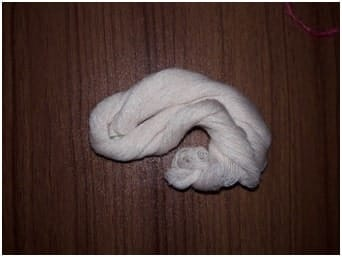Experimental Data: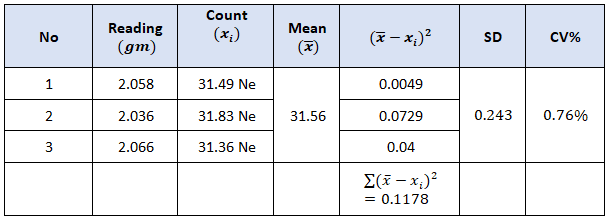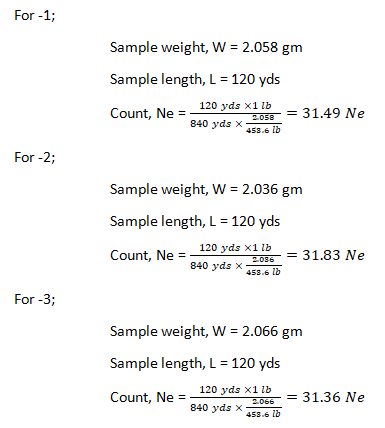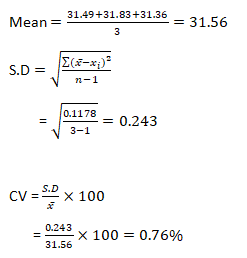Result representation: 3 sets of yarn were taken from a cotton cone package. The yarns were taken and measured in wrap reel m/c and then weight of them was taken. We got 3 different results on our samples. The count was different. The CV % was o.7 % so we can say that the yarn in the cone package were all not the same. So, the weight and count were different.

Conclusion:

From these tests we have come to learn about the ways we can measure the count for yarns. We have also learned about the processes in which we do it. I will help us in our career in the long run.